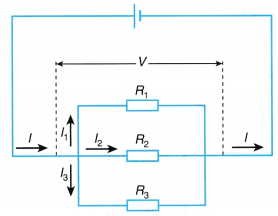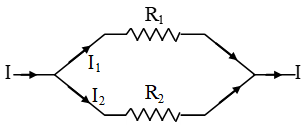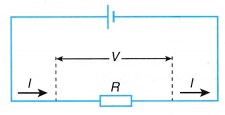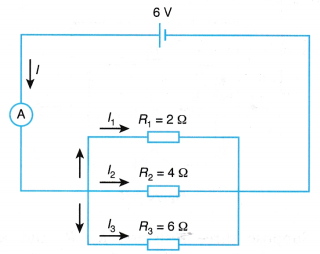# How do you calculate the total resistance of a parallel circuit?

## How do you calculate the total resistance of a parallel circuit?

The Effective Resistance of Resistors Connected in Parallel

1. There are three important characteristics in a parallel circuit:
(a) The potential difference is the same across each resistor.
(b) The current that passes through each resistor is inversely proportional to the resistance of the resistor.
(c) The total current in the circuit equals to the sum of the currents passing through the resistors in its parallel branches.2. When two or more resistances are connected between two common points so that the same potential difference is applied across each of them, they are said to be connected is parallel.When such a combination of resistance is connected to a battery, all the resistances have the same potential difference across their ends.
3. Derivation of mathematical expression of parallel combination:
Let, V be the potential difference across the two common points A and B. Then, from Ohm’s law
Current passing through R1,     I1 = V/R1             … (i)
Current passing through R2,     I2 = V/R2           … (ii)
Current passing through R3,     I3 = V/R3          … (iii)
4. If R is the equivalent resistance, then from Ohm’s law, the total current flowing through the circuit is given by,
I = V/R                               … (iv)
and I = I1 + I2 + I3           … (v)
5. Substituting the values of I, I1, I2 and I3 in Eq. (v),
$$\frac{\text{V}}{\text{R}}=\frac{\text{V}}{{{\text{R}}_{\text{1}}}}+\frac{\text{V}}{{{\text{R}}_{\text{2}}}}+\frac{\text{V}}{{{\text{R}}_{\text{3}}}}\text{ }……..\text{ (vi)}$$
6. Cancelling common V term, one gets
$$\frac{\text{1}}{\text{R}}=\frac{\text{1}}{{{\text{R}}_{\text{1}}}}+\frac{\text{1}}{{{\text{R}}_{2}}}+\frac{\text{1}}{{{\text{R}}_{3}}}$$
The equivalent resistance of a parallel combination of resistance is less than each of all the individual resistances.
7. The equivalent circuit is shown in Figure.1.  Total current through the circuit is equal to the sum of the currents flowing through it.
2.  In a parallel combination of resistors the voltage (or potential difference) across each resistor is the same and is equal to the applied voltage i.e. V1 = V2 = V3 = V.
3.  Current flowing through each resistor is inversely proportional to its resistances, thus higher the resistance of a resistors, lower will be the current flowing through it.